# Robust Direct Power Control of a DFIG Fed by a Five- Level NPC Inverter Using Neural SVPWM Technique

Robust Direct Power Control of a DFIG Fed by a Five- Level NPC Inverter Using Neural SVPWM Technique
Labo. LAAS, Departement de Génie Électrique, ENPO-MA, Oran, Algeria

Corresponding Author Email:
habib_benbouhenni@yahoo.com
Page:
119-128
|
DOI:
https://doi.org/10.18280/ti-ijes.650118
21 October 2020
|
Accepted:
10 December 2020
|
Published:
31 March 2021
| Citation

OPEN ACCESS

Abstract:

The work presents a new direct power command (DPC) strategy based on a second order sliding mode controller (SOSMC) of a doubly fed induction generator (DFIG) integrated in a wind energy conversion system (WECS). In the first step we propose to use a five-level inverter based on the neural space vector pulse width modulation (NSVPWM) to supply the DFIG rotor side. This is the harmonic distortion (THD) of the DFIG rotor voltage and then performs provides the power to the grid by the stator side. The traditional DPC with space vector pulse width modulation (DPC-SVPWM) using proportional-integral (PI) controllers has considerable reactive and active power oscillations at a steady-state operation. In order to ensure a robust DFIG DPC-SVPWM technique and minimize the reactive and active power ripples, a SOSMC algorithms is used in the second step. The Simulation results show the efficiency of the designed control scheme especially in terms of the quality of the provided power compared to DPC-SVPWM.

Keywords:

DFIG, WECS, SOSMC, DPC-SVPWM, PI, NSVPWM

1. Introduction

Classical SVPWM technique have been widely used for command of AC machine. This strategy minimizes the THD value of stator current/voltage of AC machines relative to traditional pulse width modulation (PWM). But this technique is difficult to implement compared to traditional PWM technique . the author  proposes a novel SVPWM scheme to control the two-level inverter. This proposed technique is easy and simple technique compared to classical SVPWM strategy. In addition, this designed strategy based on calculate the minimum (Min) and maximum (Max) of the three-phase voltages. The principal disadvantage of the proposed and traditional technique of SVPWM technique is the THD of stator voltage/current . In order to overcome the disadvantages of the SVPWM, intelligent SVPWM has been presented [4-6], in the traditional SVPWM, three hysteresis comparators are used to generate switching converter. Nevertheless, a few drawbacks limit the use of these comparators, such as torque oscillation and variable switching frequency of DFIGURE , the authors proposed the use of a SVPWM with artificial neural network (ANN) controllers applied to the DFIG-based wind turbine. Two-level SVPWM technique and fuzzy controller are combined to command DFIG-based wind turbine .

Vector command (VC) using traditional proportional- integral (PI) regulators is the conventional command strategy used for DFIG-based wind energy conversion system. This command strategy gives more THD of current and power oscillation. Moreover, the vector command strategy needs accurate values of the DFIG parameters and rotor speed .

In order to overcome the disadvantages of the classical vector command, direct power command (DPC) has been presented [10-12]. This technique is similar to direct torque command strategy. In DPC strategy, two hysteresis controllers, namely stator active and reactive hysteresis controllers are selected to determine the inverter instantaneous switching state. The instantaneous switching state of the rotor side converter is determined based on the stator reactive and stator active power errors. DPC technique based on an estimated stator flux has been proposed . DPC technique was designed based on stator flux orientation command scheme with a constant switching frequency , where a reference rotor voltage was calculated based on the estimated stator flux, active and reactive powers and their errors. An stator  active and reactive power traditional PI controllers and SVPWM were combined to replace the conventional hysteresis comparators. The DPC technique of a wind turbine driven DFIGs connected to distorted grid voltage conditions was presented . A DPC strategy was proposed based on neural algorithm to minimizes the THD value of stator current/voltage of DFIG supplied by five-level NPC inverter . A seven-level DPC control scheme with SVPWM technique was proposed to reduce the reactive and active power oscillations . Two second order sliding mode (SOSMC) controllers were proposed to regulate the reactive and active powers of DFIGURE . Direct torque control technique was proposed based on adaptive-network-based fuzzy inference system (ANFIS) and super-twisting sliding mode (STSM) to minimize the torque ripple and rotor flux ripple of DFIGURE . The neural DPC method based on neural PWM technique reduce the THD value of stator current compared to traditional DPC method of DFIGURE . The twelve sectors DPC strategy was proposed based on neural hysteresis controllers , where the traditional hysteresis comparators of DPC method is replaced by neural networks algorithms and this proposed DPC method minimize the powers ripples of DFIGURE Neural SOSMC method was proposed to control the DFIG using ANFIS-SVPWM technique; this proposed strategy is simple algorithm and reduce the active and reactive powers ripples compared to traditional SOSMC method . ANFIS algorithm and SOSMC method was combined to control the DFIG using seven-level SVPWM technique . SMC method based on ANFIS algorithm was proposed to command the DFIG using two-level SVPWM technique . DPC based on ANFIS-STSM algorithm was designed to reduce the active and reactive powers ripples of DFIG . Neural SVPWM minimized the THD value of stator current compared to neural PWM technique of DFIG controlled by fuzzy sliding mode . Seven-level SVPWM technique minimized the torque ripple compared to two-level SVPWM technique of DFIG controlled by direct vector command (DVC) . DPC technique was designed based on neural STSM algorithm to reduces the reactive/active power oscillations of the DFIG using SVPWM strategy . DVC control was proposed to command the DFIG using seven-level fuzzy SVPWM strategy . DTC control based on neural PI regulators was proposed to control the DFIG . DVC control with three-level neural SVPWM technique reduce the torque ripple compared to traditional DVC control scheme . A novel DTC technique was proposed based on neural algorithm of a DFIG using seven-level hysteresis comparator of couple . DPC strategy and neural algorithm was combined to minimizes the active power and torque oscillations of the DFIG . DPC method was proposed  based on three-level SVPWM strategy, where the designed technique minimized the THD value of current and power ripple compared to conventional DPC technique of DFIGURE DTC strategy and fuzzy STSM algorithm was combined to regulate the active/reactive power and torque of DFIG . Fuzzy SVPWM technique reduce the THD value of current compared to conventional SVPWM strategy of DFIG controlled by fuzzy SOSMC method . Neural SOSMC technique reduce the torque ripple compared to neural SMC method of DFIG . In , DVC control was proposed to control the DFIG using five-level fuzzy SVPWM technique. In , a DVC technique was designed based on seven-level neural SVPWM technique to command DFIGURE DTC strategy and neural STSM algorithm was combined to reduces the reactive/active power and torque oscillations of the DFIG . In , twelve sectors DPC technique was proposed based on neural algorithm to control the DFIGURE , five-level fuzzy SVPWM technique reduce the power ripple compared to five-level neural SVPWM technique of DFIG controlled by neural SOSMC method. In , the DTC based fuzzy STSM algorithm minimized the torque ripple compared to DTC with neural STSM algorithm. In , five-level neural SVPWM technique reduce the torque and power ripple compared to two-level neural SVPWM technique of DFIG controlled by DVC control scheme. In , the DVC method based on five-level neural SVPWM strategy reduce the harmonic distortion of current compared to traditional DVC technique of DFIGURE , four-level neural SVPWM technique reduce the torque ripple compared to three-level neural SVPWM technique of DFIG controlled by indirect vector control (IVC). In , the IVC strategy based on five-level fuzzy SVPWM strategy minimize the torque and rotor flux ripples compared to traditional IVC control of DFIGURE , two-level fuzzy PWM strategy reduce the torque and rotor flux ripples compared to two-level neural SVPWM technique of DFIG controlled by neural SMC method. In , the IVC technique based on four-level fuzzy SVPWM strategy reduce the THD value of current compared to traditional IVC control of DFIGURE in , SMC control was designed based on neural algorithm to command the DFIG using two-level neural PWM strategy. In , two-level fuzzy SVPWM strategy reduce the torque and rotor flux ripples compared to two-level neural SVPWM technique of DFIG controlled by neural SOSMC method. In , the DVC technique based on four-level neural SVPWM strategy reduce the THD value of rotor/stator current and active/reactive power oscillations compared to DVC-PWM technique of DFIGURE Fuzzy SOSMC method reduce the rotor flux and torque ripples compared to fuzzy SMC control scheme of the DFIG . The IVC control scheme minimize the torque and rotor flux oscillations compared to DVC method of the DFIG . In , the IVC technique based on three-level neural SVPWM strategy minimize the torque oscillation and the harmonic distortion of rotor/stator current compared to IVC-PWM method of the DFIGURE

The original contribution of this work is the application of the STSM algorithm in the DPC method to command the active and reactive power of the DFIG drives supplied by a five-level neural NSVPWM technique. The simulation results validate that the DPC-STSM-5L-NSVPWM has very robust behavior, like traditional DPC control scheme with PI controller, and it reduces the harmonic distortion of stator current. This represent a novel robust and oscillation-free DPC technique for DFIG drive command.

2. Five-Level NSVPWM Technique

Traditionally the SVPWM technique is widely used in variable speed drive of machines, especially for VC method and DPC control. In addition, this technique is difficult to implement. But this strategy minimize the harmonic distortion. This technique gives 15% more voltage output compare to the traditional PWM strategy . Furthermore it reduces the harmonic distortion as well as loss due to reduce number of commutations in the converter.

Table 1. Parameters of the neural algorithm

 Parameters Values TrainParam.show 50 TrainParam.Lr 0.02 Number of hidden layer 1 TrainParam.mu 0.9 Coeff of acceleration of convergence (mc) 0.9 TrainParam.goal 0 TrainParam.eposh 300 Functions of activation Tensing, Purling, gensim

In this section, we propose use a five-level NPC inverter to control the rotor side converter of DFIGURE in addition, this inverter controlled by SVPWM strategy. The structure of the proposed SVPWM technique is given in Figure 1. This technique gives more THD value of current and power oscillations. The structure of hysterisis controllers is given in Figure 2.

In order to overcome the desadevantages of the five-level SVPWM technique a complimentary use of the neural algorithm is proposed. The neural SVPWM technique (NSVPWM) is a modification of the five-level SVPWM technique, where the traditional hysteresis controllers, has been replaced by a ANN controller (see Figure 3 and Figure 4). This proposed technique is easy modulation scheme and simple algorithm. In addition, this designed technique minimize the THD value of current and voltage compared to traditional SVPWM and PWM technique.

In the NSVPWM technique, the training used is that of the retropropagation of Levenberg-Marquardt (LM). The parameters of the neural algorithm is shown in Table 1. The block diagram of the ANN controller is shown in Figure 5.

## 1.png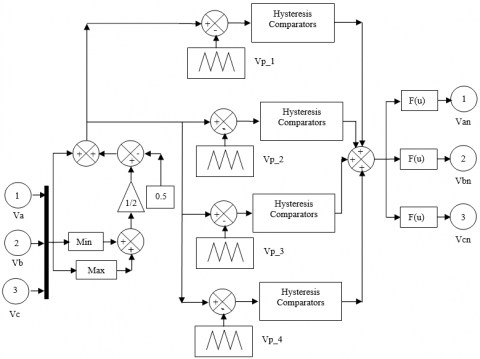Figure 1. Block diagram of five-level SVPWM

## 2.png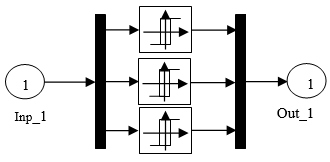Figure 2. Structure of hysteresis controllers

## 3.png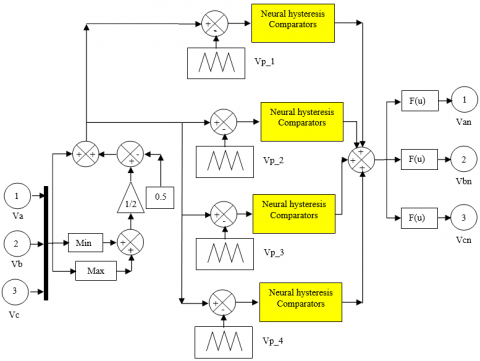Figure 3. Block diagram of five-level NSVPWM

## 4.png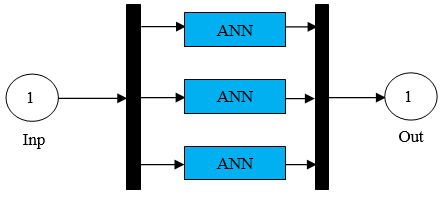Figure 4. Structure of neural hysteresis controllers

## 5.png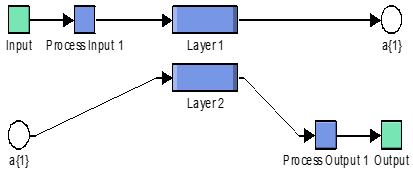Figure 5. Structure of neural controllers

3. SOSMC Method with Five-Level NSVPWM Technique

The SOSM-DPC method with five-level NSVPWM technique (DPC-SOSMC-NSVPWM) goal is to command the stator active power, and the stator reactive power magnitude of the DFIGURE the stator reactive power is regulated by means of the direct axis voltage Vdr, while the stator active power is regulated by means of the quadrature axis voltage Vqr .

The disadvantage of the DPC control scheme with PI controllers (see Figure 6) is the power oscillation and THD value of current. To minimize the power ripple . A SOSM controller and five-level NSVPWM strategy has been designed.

SOSM controller is one of the most interesting nonlinear control approaches. The second order sliding mode direct power control with five-level NSVPWM strategy (DPC-SOSMC-NSVPWM) is a modification of the DPC control scheme with PI controllers, where the classical PI controller, has been replaced by a SOSM controller and SVPWM technique are replaced by NSVPWM technique.

The proposed DPC-SOSMC-NSVPWM, which is proposed to command stator reactive and active power of the DFIG is shown in Figure 7. However, this technique is robust, easy and simple command. In addition, this technique minimize the power oscillation and THD value relative to vector control and traditional control scheme.

The SOSMC stator reactive and active power controllers are proposed to change respectively the q and d-axis voltages (Vdr and Vqr) as in Eqns. (1) and (2) .

$V_{d r}=K_{1}\left|S_{Q s}\right|^{r} \operatorname{sign}\left(S_{Q s}\right)+V_{d r 1} 1$

$\dot{V}_{d r 1}=K_{1} \operatorname{sign}\left(S_{Q s}\right)$                  (1)

$V_{q r}=K_{2}\left|S_{P_{s}}\right|^{r} \operatorname{sign}\left(S_{P_{s}}\right)+V_{q r 1}$

$\dot{V}_{q r 1}=K_{2} \operatorname{sign}\left(S_{P_{s}}\right)$         (2)

where, the flux magnitude error SQs=Qs*-Qs and the torque error SPs=Ps*- Ps are the manifolds, and the constant gains K1 and K2 must check the stability conditions.

The proposed SOSMC technique, which is proposed to regulate the active and reactive powers of the DFIG is shown in Figure 8.

## 8.png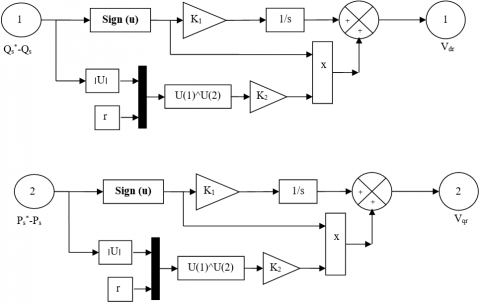Figure 6. DPC control with PI controller

4. Simulation Results

The DFIG used in this paper is a 1 MW, 380/690 V, 50 Hz, machine whose nominal parameters are reported in Table 2. The both control techniques DPC-SOSMC-NSVPWM and DPC-PI are simulated and compared in terms of reference tracking, robustness against machine parameter variations and stator current harmonics distortion. The simulations are lead with the Matlab/Simulink software.

4.1 Reference tracking test

The objective of this test is to study the behaviour of both proposed techniques while the DFIG’s speed is considered maintained at its nominal value.

Figures 9-14 show the obtained simulation results. As it’s shown by Figure 11 and 12, for the two DPC techniques, the active and reactive powers tracks well perfectly their active and reactive power references.

Moreover, contrary to the DPC-PI control scheme where the coupling effect between the two axes is apparent, we notice that the DPC-SOSMC-NSVPWM control guarantees the decoupling between them.

Figures 9-10 shows the THD value of the current of the DFIG for both DPC techniques. It can be clear observed that the THD value is minimized for DPC-SOSMC-NSVPWM technique (THD=0.43%) when compared to DPC with PI controllers (THD=1.64%).

## 7.png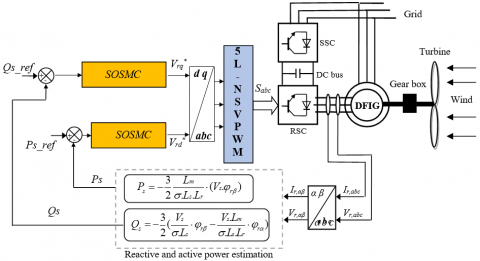Figure 7. DPC control with SOSMC and five-level NSVPWM technique

## 8.pngFigure 8. SOSMC controller

## 9.png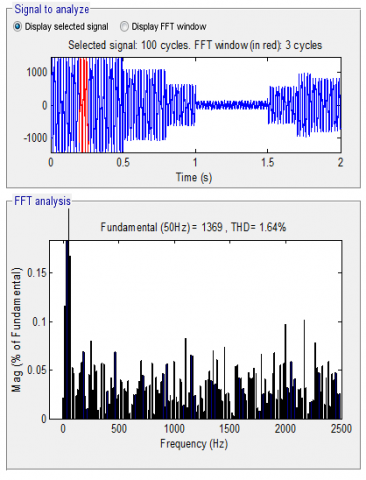Figure 9. THD of current (DPC-PI)

## 10.png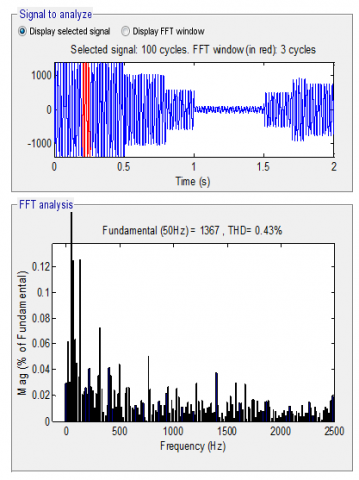Figure 10. THD of current (DPC-SOSMC-NSVPWM)

## 1.pngFigure 11. Stator active power (RTT)

## 2.png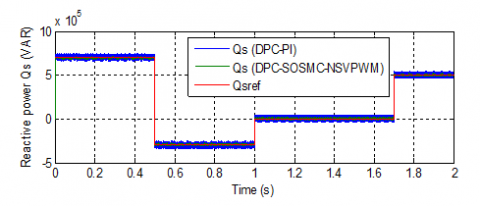Figure 12. Stator reactive power (RTT)

## 3.png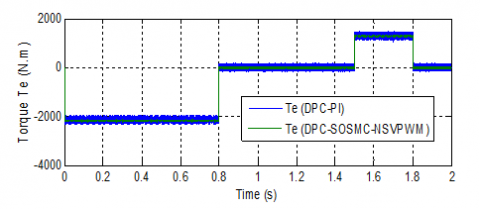Figure 13. Torque (RTT)

## 4.png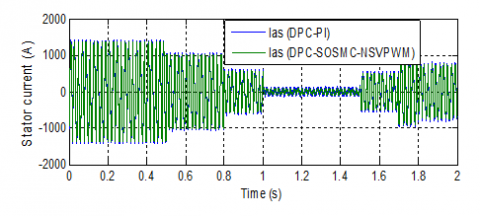Figure 14. Stator current (RTT)

## 5.png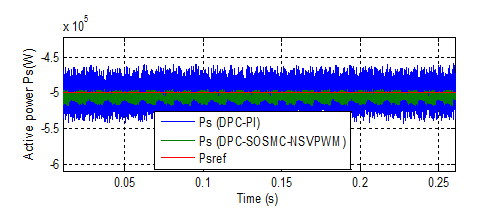Figure 15. Zoom in the active power (RTT)

## 6.png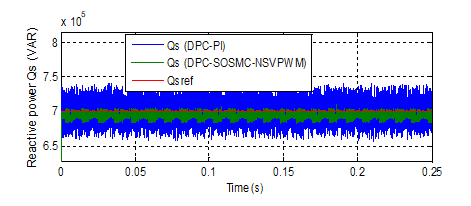Figure 16. Zoom in the stator reactive power (RTT)

## 7.png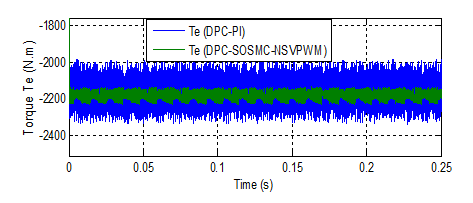Figure 17. Zoom in the torque (RTT)

## 8.png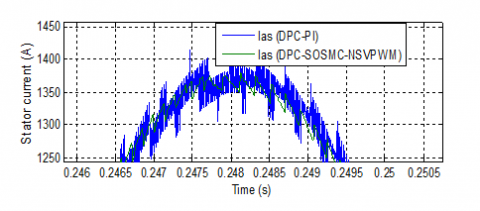Figure 18. Zoom in the current (RTT)

4.2 Robustness test

## 19.png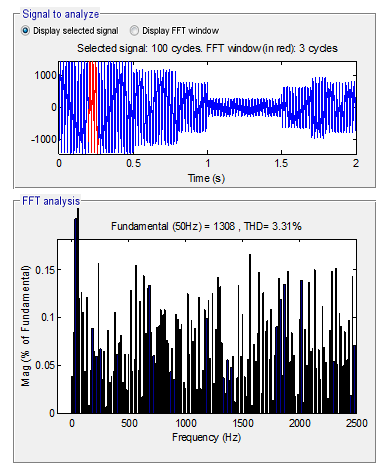Figure 19. THD of current (DPC-PI)

In this part, the values of the Rs and Rr are doubled and the values of the Ls, Lr and Lm are divided by 2. The DFIG is running at its nominal speed. Figures 19-24 shows the obtained simulation results. As its shown by these Figures, we notice that parameter variations of the DFIG increase slightly the time-response of the DPC-PI technique. This results show these variations present a clear effect on the stator current, reactive power, active power and torque curves and that the effect appears more important for the DPC-PI than that with DPC-SOSMC-NSVPWM technique. Thus it can be concluded that the proposed DPC-SOSMC-NSVPWM technique is more robust than the DPC-PI one.

## 20.png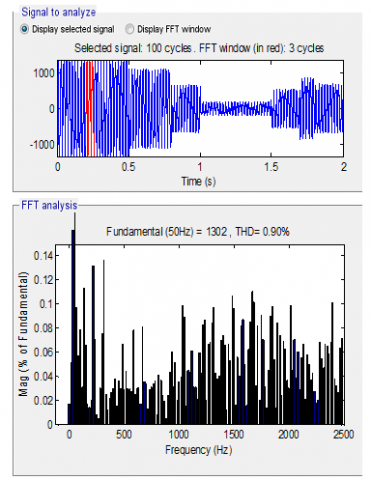Figure 20. THD of current (DPC-SOSMC-NSVPWM)

## 21.png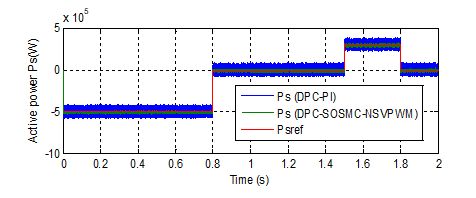Figure 21. Stator active power (RT)

## 22.png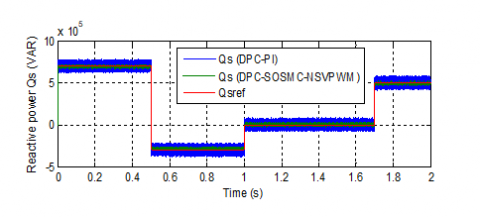Figure 22. Stator reactive power (RT)

## 23.png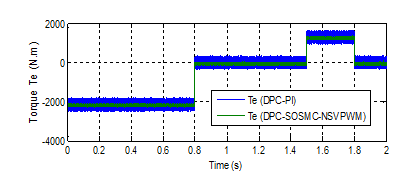Figure 23. Torque (RT)

## 24.png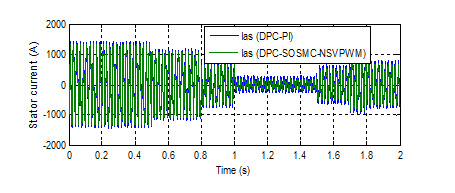Figure 24. Stator current (RT)

## 25.png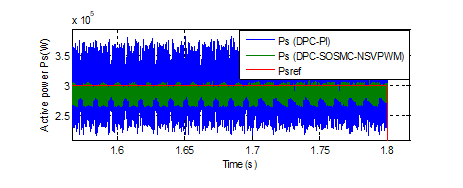Figure 25. Zoom in the stator active power (RT)

## 26.png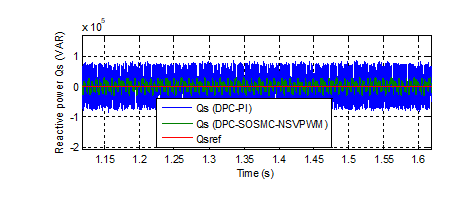Figure 26. Zoom in the reactive power (RT)

## 27.png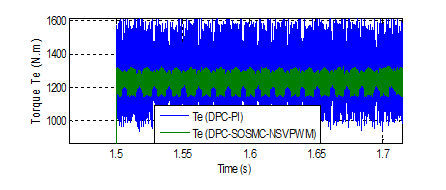Figure 27. Zoom in the torque (RT)

## 28.pngFigure 28. Zoom in the stator current (RT)

5. Conclusions

A new DPC control scheme of a DFIG connected directly to the grid by the stator side and fed by an five-level NSVPWM technique from the RSC has been presented in this work. In the first step, we started with a study of NSVPWM technique of the five-level NPC inverter. This proposed technique has many advantages as well as reduce the harmonic distortion, thereby increasing the DC bus utilization compared to traditional PWM technique.

In second step, we proposed a novel DPC technique based on SOSMC and five-level NSVPWM technique in order to command independently active and reactive powers exchanged between the DFIG and the grid. The proposed technique is synthesized and compared to the DPC control with PI regulators. In term of reference tracking with a DFIG in ideal conditions both DPC strategies track almost perfectly their references but a coupling effect is appeared in the DPC-PI responses which is eliminated in the DPC-SOSMC-NSVPWM ones. On the other hand simulation results have confirmed that the designed control scheme operates with a very lower power ripple. A robustness test has also been investigated where the DFIG parameters have been intentionally modified. These changes induce some disturbances on the stator reactive and stator active power responses but with an effect almost doubled with the DPC-PI control scheme than on that with DPC-SOSMC-NSVPWM one. Basing on all these results it can be concluded that robust technique as DPC-SOSMC-NSVPWM can be a very attractive solution for devices using DFIG such as wind turbine.

Appendix

Table 2. Parameters of the simulated DFIG

 Pn 1.5 MW p 2 Vn 380V Rr Rs Lm Lr Ls 0.021Ω 0.012Ω 0.0135H 0.0136H 0.0137H fr J 0.0024Nm.s/rad 1000 Kg.m2 f 50Hz
References

 Benbouhenni, H., Boudjema, Z., Belaidi, A. (2018). Using three-level Fuzzy space vector modulation method to improve indirect vector control strategy of a DFIG based wind energy conversion systems. International Journal of Smart Grid, 2(3): 155-171.

 Benbouhenni, H. (2018). Comparative study between direct vector control and fuzzy sliding mode controller in three-level space vector modulation inverter of reactive and active power command of DFIG-based wind turbine systems. International Journal of Smart Grid, 2(4): 188-196.

 Benbouhenni, H., Boudjema, Z., Belaidi, A. (2019). A novel matlab/simulink model of DFIG drive using NSMC method with NSVM strategy. International Journal of Applied Power Engineering (IJAPE), 8(3): 221-233.

 Benbouhenni, H., Boudjema, Z., Belaidi, A. (2019). Direct vector control of a DFIG supplied by an intelligent SVM inverter for wind turbine system. Iranian Journal of Electrical & Electronic Engineering, 15(1): 45-55.

 Benbouhenni, H. (2017). Hybrid neural sliding mode control of a DFIG speed in wind turbine. Majlesi Journal of Energy Management, 6(4): 31-41.

 Benbouhenni, H., Boudjema, Z., Belaidi, A. (2018). Neuro-second order sliding mode control of a DFIG supplied by a two-level NSVM inverter for wind turbine system. Iranian Journal of Electrical and Electronic Engineering, 14(3): 362-373.

 Benbouhenni, H. (2018). Comparative study between NSVM and FSVM strategy for a DFIG-based wind turbine system controlled by neuro-second order sliding mode. Majlesi Journal of Mechatronic Systems, 7(1): 33-43.

 Benbouhenni, H., Boudjema, Z., Belaidi, A. (2019). Indirect vector control of a DFIG supplied by a two-level FSVM inverter for wind turbine system. Majlesi Journal of Electrical Engineering, 13(1): 45-54.

 Benbouhenni, H., Boudjema, Z., Belaidi, A. (2018). DFIG-based WT system using FPWM inverter. International Journal of Smart Grid, 2(3): 142-154.

 Boulahia, A., Nabti, K., Benalla, H. (2012). Direct Power Control for AC/DC/AC Converters in Doubly Fed Induction Generators Based Wind Turbine. International Journal of Electrical and Computer Engineering, 2(3): 425-432.

 Jou, S., Lee, S., Park, Y., Lee, K. (2009). Direct Power Control of a DFIG in Wind Turbines to Improve Dynamic Responses. Journal of Power Electronics, 9(5): 781-790.

 Tavakoli, S.M., Pourmina, M.A., Zolghadri, M.R. (2013). Comparison between different DPC methods applied to DFIG wind turbines. International Journal of Renewable Energy Research, 3(2): 446-452.

 Xu, L., Cartwright, P. (2006). Direct active and reactive power control of DFIG for wind energy generation. IEEE Trans. Energy Convers. 21(3): 750-758. https://doi.org/10.1109/TEC.2006.875472

 Zhi, D., Xu, L. (2007). Direct power control of DFIG with constant switching frequency an improved transient performance. IEEE Trans. Energy Convers, 22(1): 110-118.

 Raj, M.S, Saravanan, T., Srinivasan, V. (2014). A modified direct torque control of induction motor using space vector modulation technique. Middle-East Journal of Scientific Research, 20(11): 1572-1574.

 Shehata, E.G., Salama, G.M. (2013). Direct power control of DFIGs based wind energy generation systems under distorted grid voltage conditions. International Journal of Electrical Power & Energy Systems, 53: 956-966. https://doi.org/10.1016/j.ijepes.2013.06.006

 Benbouhenni, H. (2019). Application of five-level NPC inverter in DPC-ANN of doubly fed induction generator for wind power generation systems. International Journal of Smart Grid, 3(3).

 Benbouhenni, H. (2019). Direct power control of a DFIG fed by a seven-level inverter using SVM strategy. International Journal of Smart Grid, 3(2): 54-62.

 Benbouhenni, H. (2018). Fuzzy second order sliding mode controller based on three-level fuzzy space vector modulation of a DFIG for wind energy conversion systems. Majlesi Journal of Mechatronic Systems, 7(3): 17-26.

 Benbouhenni, H. (2020). Utilization of an ANFIS-STSM algorithm to minimize total harmonic distortion. International Journal of Smart Grid, 4(2): 56-67.

 Benbouhenni, H., Boudjema, Z., Belaidi, A. (2020). Power Control of DFIG in WECS Using DPC and NDPC-NPWM Methods. Mathematical Modelling of Engineering Problems, 7(2): 223-236.

 Benbouhenni, H. (2020). Twelve sectors DPC control based on neural hysteresis comparators of the DFIG integrated to wind power. Tecnica Italiana-Italian Journal of Engineering Science, 64(2): 223-236.

 Benbouhenni, H., Boudjema, Z., Belaidi, A. (2019). Higher control scheme using neural second order sliding mode and ANFIS-SVM strategy for a DFIG-based wind turbine. International Journal of Advances in Telecommunications, Electrotechnics, Signals and Systems, 8(2): 17-28.

 Benbouhenni, H. (2019). Second order sliding mode with ANFIS controllers for DFIG using seven-level NSVPWM technique. Majlesi Journal of Energy Management, 8(1): 29-39.

 Benbouhenni, H. (2020). ANFIS-sliding mode control of a DFIG supplied by a two-level SVPWM technique for wind energy conversion system. International Journal of Applied Power Engineering (IJAPE), 9(1): 36-47. https://doi.org/10.11591/ijape.v8.i1.pp36-47

 Benbouhenni, H., Boudjema, Z., Belaidi, A. (2020). DPC based on ANFIS super-twisting sliding mode algorithm of a doubly-fed induction generator for wind energy system. Journal Européen des Systèmes Automatisés, 53(1): 69-80. https://doi.org/10.18280/jesa.530109

 Benbouhenni, H., Boudjema, Z., Belaidi, A. (2020). Comparison study between NPWM and NSVPWM strategy in FSMC control of stator reactive and active powers control of a DFIG-based wind turbine system. International Journal of Applied Power Engineering (IJAPE), 9(2): 159-172. https://doi.org/10.11591/ijape.v9.i2.pp159-172

 Benbouhenni, H. (2020). Comparison study between seven-level SVPWM and two-level SVPWM strategy in direct vector control of a DFIG-based wind energy conversion systems. International Journal of Applied Power Engineering (IJAPE), 9(1): 12-21. https://doi.org/10.11591/ijape.v8.i1.pp12-21

 Benbouhenni, H., Boudjema, Z., Belaidi, A. (2021). Direct power control with NSTSM algorithm for DFIG using SVPWM technique. Iranian Journal of Electrical and Electronic Engineering, 17(1): 1518-1518.

 Benbouhenni, H. (2019). Reducing Current and Torque Ripples in DVC Control of DFIG Operation at Constant Switching Frequency for Wind Generation Application. Majlesi Journal of Energy Management, 8(4): 47-55.

 Benbouhenni, H. (2019). Torque Ripple Reduction of DTC DFIG Drive using Neural PI Regulators. Majlesi Journal of Energy Management, 8(2): 21-26.

 Benbouhenni, H., Boudjema, Z., Belaidi, A. (2018). Direct vector command based on three-level NSVM of a doubly fed induction generator for wind energy conversion. In 2018 International Conference on Applied Smart Systems (ICASS), 1-8. https://doi.org/10.1109/ICASS.2018.8651957

 Benbouhenni, H., Boudjema, Z. (2018). Two-level DTC based on ANN controller of DFIG using 7-level hysteresis command to reduce flux ripple comparing with traditional command. In 2018 International Conference on Applied Smart Systems (ICASS), 1-8. https://doi.org/10.1109/ICASS.2018.8652013

 Benbouhenni, H., Boudjema, Z., Belaidi, A. (2020). Power ripple reduction of DPC DFIG drive using ANN controller. Acta Electrotechnica et Informatica, 20(1): 15-22. https://doi.org/10.15546/aeei-2020-0003

 Benbouhenni, H. (2019). A direct power control of the doubly fed induction generator based on the three-level NSVPWM technique. International Journal of Smart Grid, 3(4): 216-225.

 Benbouhenni, H. (2019). Stator current and rotor flux ripples reduction of DTC DFIG drive using FSTSMC algorithm. International Journal of Smart Grid, 3(4): 226-234.

 Benbouhenni, H. (2019). Comparison study between SVPWM and FSVPWM strategy in fuzzy second order sliding mode control of a DFIG-based wind turbine. Carpathian Journal of Electronic and Computer Engineering, 12(2): 1-10. https://doi.org/10.2478/cjece-2019-0009

 Benbouhenni, H. (2019). A comparative study between NSMC and NSOSMC strategy for a DFIG integrated into wind energy system. Carpathian Journal of Electronic and Computer Engineering, 12(1): 1-8. https://doi.org/10.2478/cjece-2019-0001

 Benbouhenni, H., Boudjema, Z., Belaidi, A. (2020). DFIG-based wind energy conversion system using five-level FSVM technique. International Journal of Renewable Energy Technology, 11(2): 147-164. https://doi.org/10.1504/IJRET.2020.108300

 Benbouhenni, H. (2019). Application of seven-level neural space vector PWM in direct vector control system of doubly fed induction generator for wind turbine. International Journal of Smart Grid, 3(3): 163-171.

 Benbouhenni, H. (2018). Rotor flux and torque ripples minimization for direct torque control of DFIG by NSTSM algorithm. Majlesi Journal of Energy Management, 7(3): 1-9.

 Benbouhenni, H., Boudjema, Z., Belaidi, A. (2018). Sensorless twelve sectors implementation of neural DPC controlled DFIG for reactive and active powers ripples reduction. Majlesi Journal of Energy Management, 7(2).

 Benbouhenni, H. (2018). New hybrid five-level space vector modulation strategy to minimize current distortion and power ripple for a DFIG controlled by NSOSMC control. Majlesi Journal of Energy Management, 7(2): 33-44.

 Benbouhenni, H. (2018). A comparative study between DTC-NSTMC and DTC-FSTSMC control scheme for a DFIG-based wind turbine. Majlesi Journal of Energy Management, 7(4): 43-53.

 Benbouhenni, H. (2019). Direct vector control for doubly fed induction generator-based wind turbine system using five-level NSVM and two-level NSVM technique. International Journal of Smart Grid, 3(1): 25-32.

 Benbouhenni, H. (2019). Stator active and reactive power ripples minimization for DVC control of DFIG by using five-level neural space vector modulation. Acta Electrotechnica et Informatica, 19(2): 16-23.

 Benbouhenni, H., Boudjema, Z., Belaidi, A. (2018). A comparative study between four-level NSVM and three-level NSVM technique for a DFIG-based WECSs controlled by indirect vector control. Carpathian Journal of Electronic and Computer Engineering, 11(2): 13-19.

 Benbouhenni, H. (2019). Intelligence indirect vector control of a DFIG based wind turbines. Majlesi Journal of Electrical Engineering, 13(3): 27-35.

 Benbouhenni, H. (2017). Comparison study between FPWM and NSVM inverter in neuro-sliding mode control of reactive and active power control of a DFIG-based wind energy. Majlesi Journal of Energy Management, 6(4): 15-23.

 Benbouhenni, H., Boudjema, Z., Belaidi, A. (2017). DFIG-based wind turbine system using four-level FSVM strategy. Majlesi Journal of Energy Management, 6(3): 7-19.

 Benbouhenni, H. (2019). Sliding mode with neural network regulateur for DFIG using two-level NPWM strategy. Iranian Journal of Electrical & Electronic Engineering, 15(3): 411-419.

 Benbouhenni, H. (2018). Comparative study between NSVM and FSVM strategy for a DFIG-based wind turbine system controlled by neuro-second order sliding mode. Majlesi Journal of Mechatronic Systems, 7(1): 33-43.

 Benbouhenni, H., Boudjema, Z., Belaidi, A. (2019). Using four-level NSVM technique to improve DVC control of a DFIG based wind turbine systems. Periodica Polytechnica Electrical Engineering and Computer Science, 63(3): 144-150.

 BENBOUHENNI, H. (2019). A comparative study between FSMC and FSOSMC strategy for a DFIG-based wind turbine system. Majlesi Journal of Mechatronic Systems, 8(2): 7-14.

 Benbouhenni, H. (2018). Comparative study between different vector control methods applied to DFIG wind turbines. Majlesi Journal of Mechatronic Systems, 6(4): 15-23.

 Benbouhenni, H., Boudjema, Z., Belaidi, A. (2018). DFIG-based wind turbine system using three-level neural space vector modulation technique. Majlesi Journal of Mechatronic Systems, 7(2): 35-45.

 Benbouhenni, H. (2019). A comparison study between fuzzy PWM and SVM inverter in NSMC control of stator active and reactive power control of a DFIG based wind turbine systems. International Journal of Applied Power Engineering (IJAPE), 8(1): 78-92. https://doi.org/10.11591/ijape.v8.i1.pp78-92

 Bouyekni, A., Taleb, R., Boudjema, Z., Kahal, H. (2018). A second-order continuous sliding mode based on DPC for wind-turbine-driven DFIG. Elektrotehniski Vestnik, 85(1/2): 29-36.

 Benbouhenni, H. (2018). Neuro-sconde order sliding mode field oriented control for DFIG based wind turbine. International Journal of Smart Grid, 2(4): 209-217.